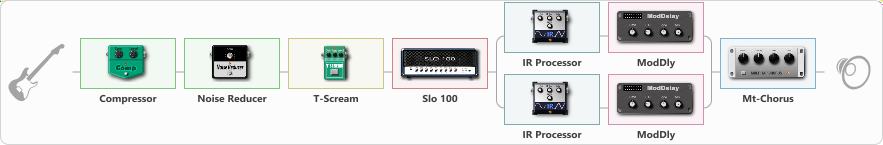# Michal My Solo

Discussion in 'ToneLib-GFX presets' started by Michael309, May 27, 2023.

1. Michal My Solo

Effects chain:Effect: "Compressor" (Dynamics / Filter), active - "yes"
{
"Sense" = 21
"Level" = 50
}

Effect: "Noise Reducer" (Dynamics / Filter), active - "yes"
{
"Sens" = 88
"Mode" = Soft
}

Effect: "T-Scream" (Overdrive / Distortion), active - "yes"
{
"Drive" = 28
"Tone" = 36
"Level" = 100
}

Effect: "Slo 100" (Amp simulators), active - "yes"
{
"Gain" = 60
"Bass" = 51
"Middle" = 80
"Treble" = 56
"Presence" = 51
"Master" = 82
"Level (dB)" = 0
}

Effect: "Splitter" (Dynamics / Filter)
{
"A-Bypass" = Off
"A-Pan" = -64
"A-Level" = 55
"B-Bypass" = Off
"B-Pan" = 61
"B-Level" = 55

'A' branch:
{

Effect: "IR Processor" (Cabinets), active - "yes"
{
"IR" = IR -DO 4x12-SM57 Rhythm
"Low Cut (Hz)" = 99
"Hi Cut (kHz)" = 8.5
"Mix" = 100
"Level (dB)" = 0
}

Effect: "ModDly" (Delay), active - "yes"
{
"Time" = 600
"Feedback" = 35
"Tone" = 70
"Speed" = 1.7
"Mix" = 50
}
}
'B' branch:
{

Effect: "IR Processor" (Cabinets), active - "yes"
{
"IR" = IR -DO 4x12-SM57 Rhythm
"Low Cut (Hz)" = 81
"Hi Cut (kHz)" = 8.5
"Mix" = 100
"Level (dB)" = 0
}

Effect: "ModDly" (Delay), active - "yes"
{
"Time" = 400
"Feedback" = 35
"Tone" = 70
"Speed" = 1.7
"Mix" = 50
}
}
}

Effect: "Mt-Chorus" (Modulation / Sfx), active - "yes"
{
"Speed" = 1.9
"Depth" = 32
"Time" = 4.8
"Mix" = 28
}

Note: You will need to download and install the ToneLib-GFX software to use the preset.

#### Attached Files:

• ###### Michal_My_Solo.tlgfx
File size:
27.1 KB
Views:
2,609
Armandoyvxn and Grac like this.